# Standard enthalpy of solution

Chemical Thermodynamics: Standard enthalpy of solution

A solution is defined as a homogeneous mixture of two or more substances in their gas, liquid or solid phase. The enthalpy of solution is defined as the amount of heat absorbed or evolved by a solution to dissolve its solutes in the solvent at constant pressure.

The formation of a solution requires the formation for ions from its solute as well as solvent. Here, the solvent is the substance that is greater in quantity. The dissolution of a solid in a solvent can be described as follows:

Solute(s) + Solvent(l) –> Solution (l)

This formation of ions may require or release energy. These ionic reactions play an important role in solution chemistry. Likewise,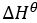Breaking up of solute and solvent into their ions is an endothermic reaction which means that is always positive for these substances separately. When the two components are mixed, a reaction between these ions may take place. The heat associated with this reaction may be endothermic or exothermic. Hence the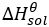can either be positive or negative depending on the nature of the reaction and the intermolecular strengths.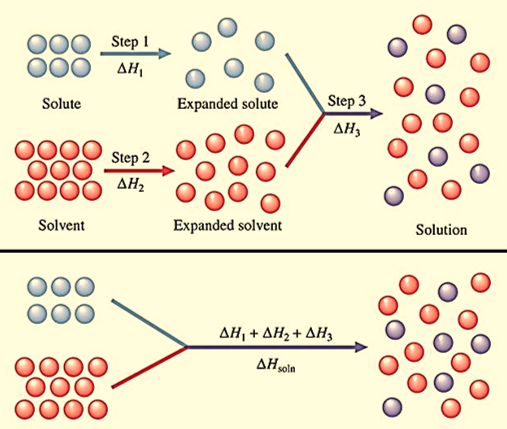Figure 1: Three step dissolution process. 1. Breaking of bonds (electrostatic interactions) in the solute (∆H1). 2. Breaking of intermolecular forces (hydrogen bonds) in the solvent(∆H2). 3. formation of solute-solvent bonds in the solution(∆H3)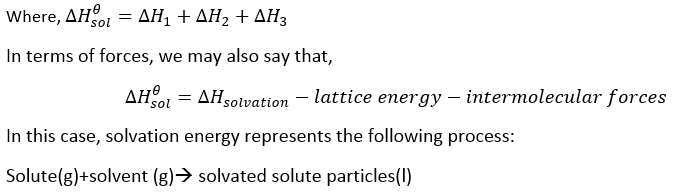If a solution is ideal, then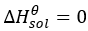. In this case, the forces of attraction between and solute and solvent particles is equal to the forces of attraction solute-solute and solvent-solvent interactions. In a non-ideal solution, these enthalpies are not equal which causes the reaction to turn either endothermic or exothermic in nature.

The standard enthalpy of solution is measured for 1 mol of the solution and the units are expressed in kJ/mol and it is measured in standard pressure of 1 atm.

When salts are dissolved in water, there is often a change in temperature due to the dissolution process. Some salt dissolves, releasing heat in the process. This is an endothermic reaction. Other reactions may dissolve while absorbing heat.In the case of salt, energy is required to break apart the hydrogen bonding between water molecules and electrostatic interactions between sodium and chloride ions. The addition of these energies is greater than the energy evolved when new solute-solvent interactions are formed between water molecules and the aqueous ions in the solution.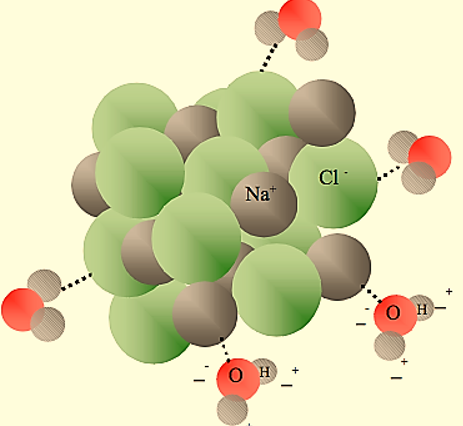Figure : Dissolution of NaCl in water. Dashed lines denote the solute-solvent interactions.

Alternatively, dissolution of potassium hydroxide in water is an exothermic reaction. In this case, more energy is released with the formation of solute-solvent bonds in comparison to the energy required for breaking the hydrogen bonds in water and ionic bonds in KOH.

As per Hess’s Law enthalpy of solution can also be represented as the sum of lattice enthalpy and hydration enthalpy: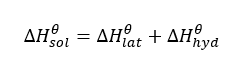For example, the enthalpy cycle for the dissolution of sodium hydroxide is as follows: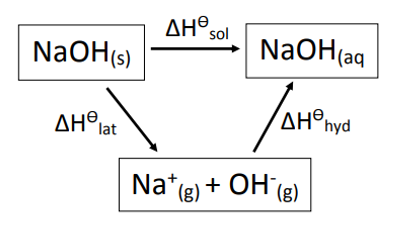Figure : Enthalpy cycle of NaOH